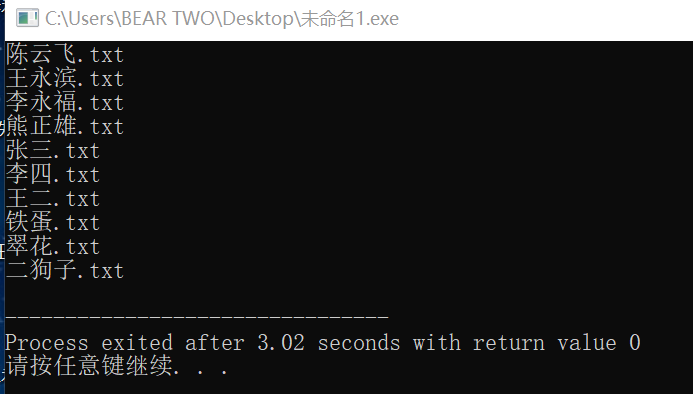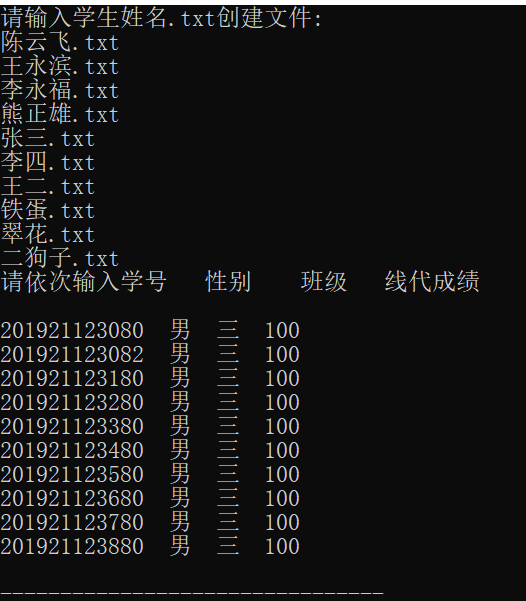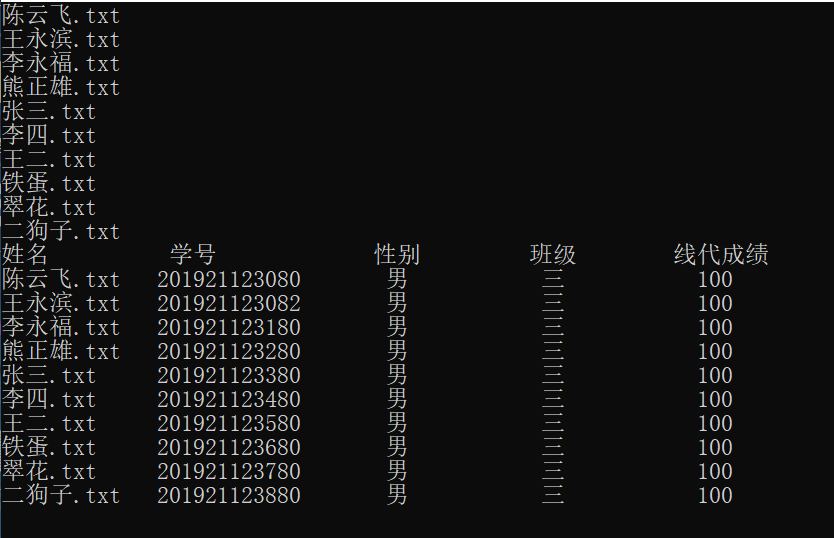# 任务一：

#include <stdio.h>
#include <stdlib.h>
int main()
{
int i;
FILE *fp;
char name;
for (i=0; i<10; i++) {
scanf("%s",name[i]);
}
for (i=0; i<n; i++) {
if ((fp = fopen (name[i],"w")) == NULL) {
printf ("File open error! \n");
exit (0);
}
}
return 0;
}# 任务二：

#include <stdio.h>
#include <string.h>
#include <stdlib.h>
struct student {
char name;
char number;
char sex;
char Class;
int score;
};
int main()
{
int i;
FILE *fp;
struct student stu;
char student;
printf ("请输入学生姓名.txt创建文件: \n") ;
for (i=0; i<10; i++) {
scanf ("%s",student[i]);
}
printf ("请依次输入学号   性别    班级   线代成绩 \n");
for (i=0; i<10; i++) {
if ((fp = fopen (student[i],"w")) == NULL) {
printf ("File open error! \n");
exit (0);
}
scanf ("%s  %s  %s  %d",stu[i].number,stu[i].sex,stu[i].Class,&stu[i].score);
fprintf(fp,"%s  %s  %s  %d",stu[i].number,stu[i].sex,stu[i].Class,stu[i].score);
fclose (fp);
}
return 0;
}# 任务三：

[要求使用结构体数组，结构体需要包含姓名，学号，性别，班级，线代成绩5个属性]

#include <stdio.h>
#include <string.h>
#include <stdlib.h>
struct student {
char name;
char number;
char sex;
char Class;
int score;
};
int main()
{
int i;
FILE *fp;
struct student stu;
char student;
for (i=0; i<10; i++) {
scanf ("%s",student[i]);
}
for (i=0; i<10; i++) {
if ((fp = fopen (student[i],"r")) == NULL) {
printf ("File open error! \n");
exit (0);
}
fscanf(fp,"%s  %s  %s  %d",stu[i].number,stu[i].sex,stu[i].Class,&stu[i].score);
fclose (fp);
}
printf ("姓名          学号             性别         班级        线代成绩 \n");
for (i=0; i<10; i++) {
printf ("%-13s%-19s%-13s%-13s%-13d\n",student[i],stu[i].number,stu[i].sex,stu[i].Class,stu[i].score);
}
return 0;
}# 任务四：

【想找几个是几个】

1、用 system("color 0A"); 其中color后面的0是背景色代号，A是前景色代号。各颜色代码如下：

0=黑色 1=蓝色 2=绿色 3=湖蓝色 4=红色 5=紫色 6=黄色 7=白色 8=灰色 9=淡蓝色 A=淡绿色 B=淡浅绿色 C=淡红色 D=淡紫色 E=淡黄色 F=亮白色。

2、 system（“ATTRIB”）显示或更改文件属性 。

3、system("cls")\\清屏。

4、system("pause")\\暂停，按任意键继续。

posted @ 2019-12-21 16:53  熊正雄  阅读(170)  评论(0编辑  收藏  举报